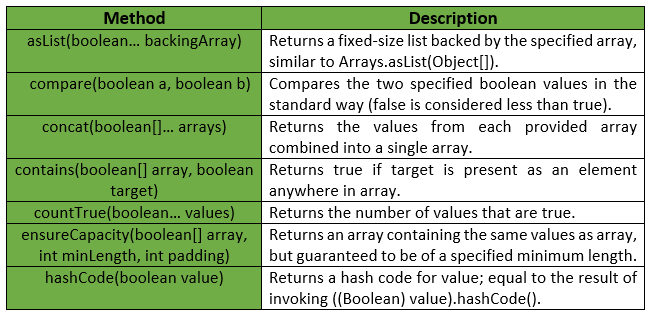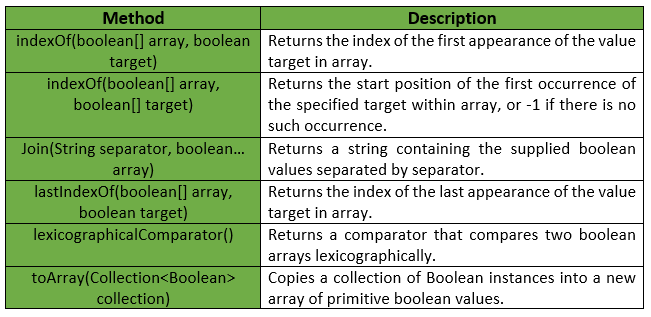# Booleans Class | Guava | Java

Booleans is a utility class for primitive type Boolean. It provides Static utility methods pertaining to boolean primitives, that are not already found in either Boolean or Arrays.

Declaration :

```@GwtCompatible(emulated=true)
public final class Booleans
extends Object
```

Below given are some methods provided by Guava Booleans Class :Exceptions :

• ensureCapacity : IllegalArgumentException if minLength or padding is negative.
• toArray : NullPointerException if collection or any of its elements is null.

Following table shows some other methods provided by Guava Booleans Class :Below given are some examples showing the implementation of Guava Booleans Class methods :
Example 1 :

 `// Java code to show implementation ` `// of Guava Booleans.asList() method ` ` `  `import` `com.google.common.primitives.Booleans; ` `import` `java.util.*; ` ` `  `class` `GFG { ` `    ``// Driver method ` `    ``public` `static` `void` `main(String[] args) ` `    ``{ ` `        ``boolean` `arr[] = { ``true``, ``false``, ``true``, ``false``, ``true` `}; ` ` `  `        ``// Using Booleans.asList() method which ` `        ``// converts array of primitives to array of objects ` `        ``List myList = Booleans.asList(arr); ` ` `  `        ``// Displaying the elements ` `        ``System.out.println(myList); ` `    ``} ` `} `

Output :

```[true, false, true, false, true]
```

Example 2 :

 `// Java code to show implementation ` `// of Guava Booleans.toArray() method ` ` `  `import` `com.google.common.primitives.Booleans; ` `import` `java.util.*; ` ` `  `class` `GFG { ` `    ``// Driver method ` `    ``public` `static` `void` `main(String[] args) ` `    ``{ ` `        ``List myList = Arrays.asList(``true``, ``false``, ``true``, ``false``, ``true``); ` ` `  `        ``// Using Booleans.toArray() method which ` `        ``// converts a List of Booleans to an ` `        ``// array of boolean ` `        ``boolean``[] arr = Booleans.toArray(myList); ` ` `  `        ``// Displaying the elements ` `        ``System.out.println(Arrays.toString(arr)); ` `    ``} ` `} `

Output :

```[true, false, true, false, true]
```

Example 3 :

 `// Java code to show implementation ` `// of Guava Booleans.concat() method ` ` `  `import` `com.google.common.primitives.Booleans; ` `import` `java.util.*; ` ` `  `class` `GFG { ` `    ``// Driver method ` `    ``public` `static` `void` `main(String[] args) ` `    ``{ ` `        ``boolean``[] arr1 = { ``true``, ``false``, ``true` `}; ` `        ``boolean``[] arr2 = { ``false``, ``true` `}; ` ` `  `        ``// Using Booleans.concat() method which ` `        ``// combines arrays from specified ` `        ``// arrays into a single array ` `        ``boolean``[] arr = Booleans.concat(arr1, arr2); ` ` `  `        ``// Displaying the elements ` `        ``System.out.println(Arrays.toString(arr)); ` `    ``} ` `} `

Output :

```[true, false, true, false, true]
```

Example 4 :

 `// Java code to show implementation ` `// of Guava Booleans.contains() method ` ` `  `import` `com.google.common.primitives.Booleans; ` ` `  `class` `GFG { ` `    ``// Driver method ` `    ``public` `static` `void` `main(String[] args) ` `    ``{ ` `        ``boolean``[] arr = { ``true``, ``false``, ``true``, ``false``, ``true` `}; ` ` `  `        ``// Using Booleans.contains() method which ` `        ``// checks if element is present in array ` `        ``// or not ` `        ``System.out.println(Booleans.contains(arr, ``true``)); ` `        ``System.out.println(Booleans.contains(arr, ``false``)); ` `    ``} ` `} `

output :

```true
true
```

Example 5 :

 `// Java code to show implementation ` `// of Guava Booleans.compare() method ` ` `  `import` `com.google.common.primitives.Booleans; ` ` `  `class` `GFG { ` `    ``// Driver method ` `    ``public` `static` `void` `main(String[] args) ` `    ``{ ` `        ``// To compare true vs true ` `        ``System.out.println(Booleans.compare(``true``, ``true``)); ` `    ``} ` `} `

Output :

```0
```

Example 6 :

 `// Java code to show implementation ` `// of Guava Booleans.indexOf() method ` ` `  `import` `com.google.common.primitives.Booleans; ` ` `  `class` `GFG { ` `    ``// Driver method ` `    ``public` `static` `void` `main(String[] args) ` `    ``{ ` `        ``boolean``[] arr = { ``true``, ``false``, ``true``, ``false``, ``true` `}; ` ` `  `        ``// To print index of first occurence of false ` `        ``System.out.println(Booleans.indexOf(arr, ``false``)); ` `    ``} ` `} `

Output :

```1
```

Attention reader! Don’t stop learning now. Get hold of all the important Java and Collections concepts with the Fundamentals of Java and Java Collections Course at a student-friendly price and become industry ready.

My Personal Notes arrow_drop_upIn love with a semicolon because sometimes i miss it so badly)

If you like GeeksforGeeks and would like to contribute, you can also write an article using contribute.geeksforgeeks.org or mail your article to contribute@geeksforgeeks.org. See your article appearing on the GeeksforGeeks main page and help other Geeks.

Please Improve this article if you find anything incorrect by clicking on the "Improve Article" button below.

Article Tags :
Practice Tags :

Be the First to upvote.

Please write to us at contribute@geeksforgeeks.org to report any issue with the above content.